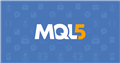# CopyBuffer function( with Handle in MutilTimeframe) not work when prev_calculated =0

CopyBuffer function not work when prev_calculated =0 and Handle in other TimeFrame which different Current TimeFrame

First I creat Indicator with two Handle in two timeframe: one in Curent TF exp: H1 and one in Orther TF exp: H4

```   Handle_Current_TF = iMA(_Symbol, _Period , 14,0,MODE_SMA,PRICE_CLOSE);
Handle_Orther_TF  = iMA(_Symbol, PERIOD_H4 , 14,0,MODE_SMA,PRICE_CLOSE); ```

Then I use CopyBuffer in OnCaculator funtion

```     int k1 =  CopyBuffer(Handle_Current_TF, 0, 1, 2, GetBuff_Cur);
int k2 =  CopyBuffer(Handle_Orther_TF,  0, 1, 2, GetBuff_Ort);
Print("prev_calculated = " , prev_calculated , " k1 = " , k1 , " k2=  " , k2);```

I Backtest this Indicator in timeframe = H1 and receive result :

```2021.03.07 12:27:06.056 2020.05.05 00:00:00   prev_calculated = 0 k1 = 2 k2=  -1
2021.03.07 12:27:06.246 2020.05.05 00:00:00   prev_calculated = 8299 k1 = 2 k2=  2
```

CopyBuffer with handle TimeFrame= H4 not work when prev_calculated =0. It mean need at least 2 tick for it to work.

This makes it difficult for me , I add Indicator to chart at weekend (market close) , Oncaculator funtion only have 1 tick.

how to solve this problem? thank for help

Files:

In fact, the number of entries to OnCalculate is not regulated. Maybe one, maybe three :).

Here is an example when there are two entries and you still do not receive information, since the indicators are not ready:

```2021.03.07 08:18:22.646 test-indi1 (XAUUSD,M5)  prev_calculated = 0 k1 = 2 k2=  -1
2021.03.07 08:18:22.646 test-indi1 (XAUUSD,M5)  prev_calculated = 50000 k1 = 2 k2=  -1
```

In fact, the number of entries to OnCalculate is not regulated. Maybe one, maybe three :).

Here is an example when there are two entries and you still do not receive information, since the indicators are not ready:

I have never seen three or more :).
Do you have any idea to solve it ? We need it work in first time Oncaculate called.

Nguyen Tien Duong :
I have never seen three or more :).
Do you have any idea to solve it ? We need it work in first time Oncaculate called.

You need? You cannot demand, so you simply cannot know anything in advance - for example, there was a swap of history. You will find out about this post factum - by the OnCalculate function (prev_calculated == 0).

You can always drop the indicator and then manually update the chart (through the right mouse click).

OnCalculateDocumentation on MQL5: Language Basics / Functions / Event Handling Functions
• www.mql5.com
Event Handling Functions - Functions - Language Basics - MQL5 Reference - Reference on algorithmic/automated trading language for MetaTrader 5
Reason: